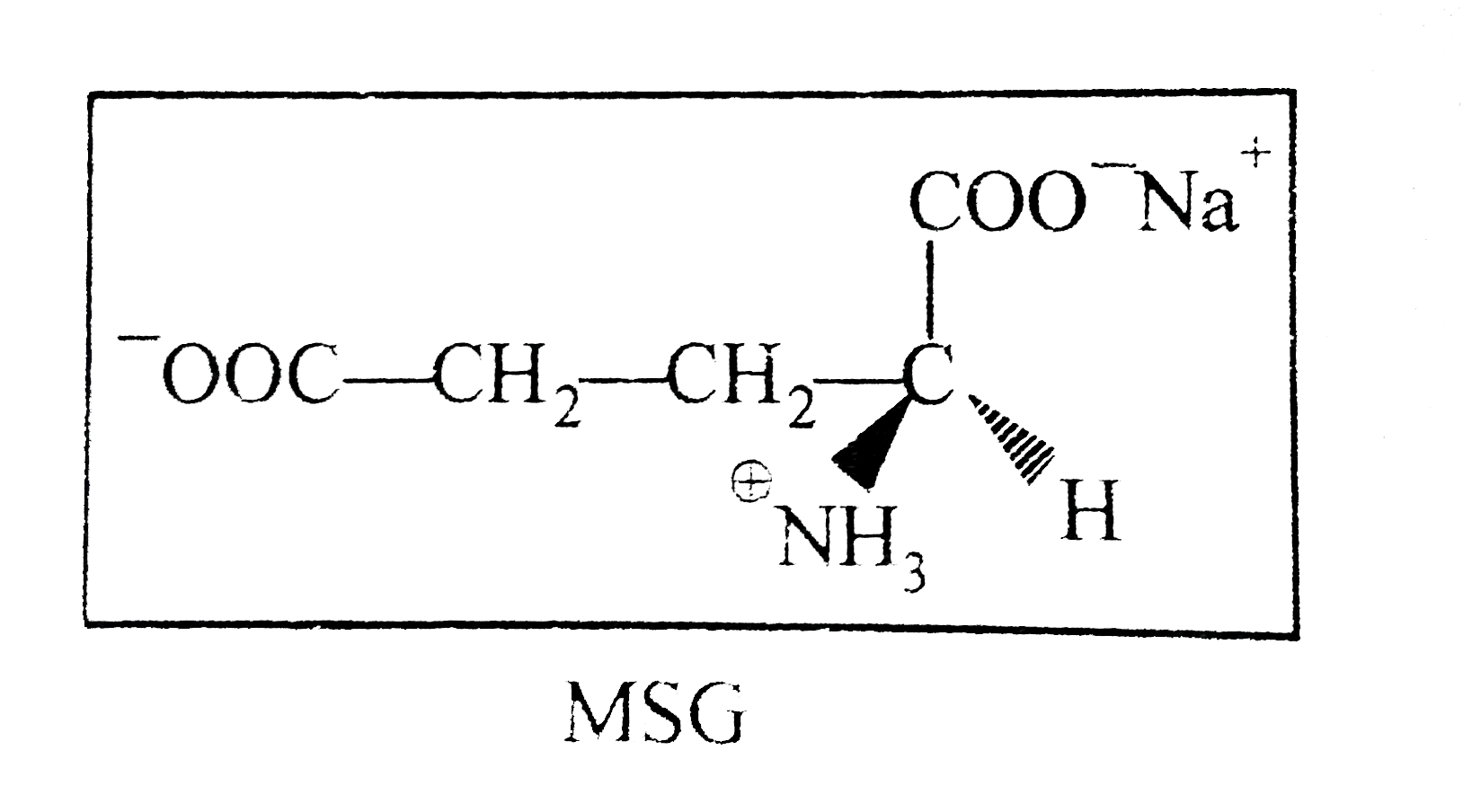# S(+) Mono Sodium Glutamate (MSG) is a flavour enhancer used in many foods. Fast foods often contain substantial amount of MSG and is widely used in Ch

590 views

closed
S(+) Mono Sodium Glutamate (MSG) is a flavour enhancer used in many foods. Fast foods often contain substantial amount of MSG and is widely used in Chines food. If one mole of above MSG was placed in 845 ml solution and passed through 200 mm tube, the observed rotation was found to be +9.6^(@).Find out of the specific rotation of (-) MSG :
A. +24^(@)
B. +56.8^(@)
C. -48^(@)
D. none of these

by (65.9k points)
selected

[Specific rotation of (-) MSG
C=(169 gm)/(845 ml) l =2m
[theta^(@)]=(theta)/(Cl)=9.6/(169//845xx2) =[-24^(@)]